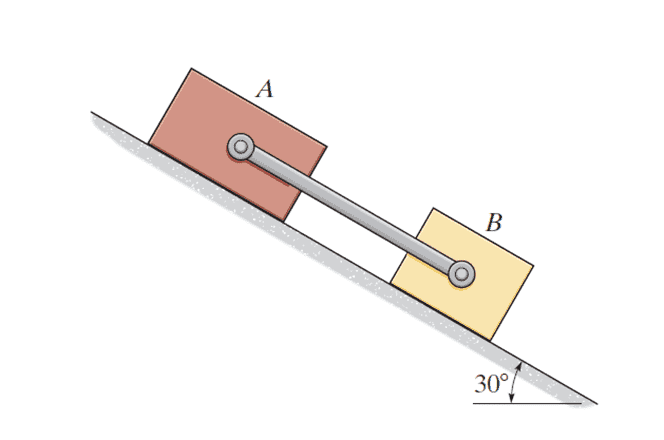# Friction Coefficients: Determining Force Direction?

Homework Statement
Blocks A and B of mass 13 kg and 10 kg respectively, are placed on the inclined plane and released. The coefficients of kinetic friction between the blocks and the inclined plane are μA=0.2 and μB=0.5 . Neglect the mass of the link.

Determine the magnitude of the force developed in the link.
Relevant Equations
nothing special.
Do I determine this from the friction coefficients?
Such that because mu_b > mu_a I know that it'll push on B because it has a bigger friction coefficient.
such that this has nothing to do with the mass of the blocks? or does it?

f.e. can I take it to be in an arbitrary direction and then solve the equations of motion for both and find the magnitude of the force in the link that way?
I did it but I might've ran an error in the analysis. I did it the other way around aka looking at it as mu_b>mu_a such that it pushes B forward and A backwards and I got the correct answer.
appreciated.What do you know about friction on an inclined plane? You have a formula for it?

•What do you know about friction on an inclined plane? You have a formula for it?
sure thing.
for B ##F_b = 42.48n##
for A ##F_a = 22.1n##
and this concludes that it will push on B and not pull it gadaimm

Where are your free body diagrams?

Last edited:
•malawi_glenn
If you are asking if you can just compare the coefficients of friction for this type of problem, it does appear to be that way. If block ##A## has the lower COF it will push on block ##B## putting the link in compression. If block ##B## has the lower COF it will pull on block ##A## putting the link in tension. Thats seems generally true for this specific type of problem ( I wasn't aware of this "shortcut" before you suggested it though).

Last edited:
can I take it to be in an arbitrary direction and then solve the equations of motion for both and find the magnitude of the force in the link that way?
Yes, you should not need to predict the direction of force, but finding the magnitude won't do it since you will need the sign to tell you which way it acts. You probably didn't mean that.
Please post that attempt if you'd like to find where you went wrong.